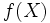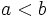# Connectedness is continuous image-closed

This article gives the statement, and possibly proof, of a topological space property (i.e., connected space) satisfying a topological space metaproperty (i.e., continuous image-closed property of topological spaces)
View all topological space metaproperty satisfactions | View all topological space metaproperty dissatisfactions
Get more facts about connected space |Get facts that use property satisfaction of connected space | Get facts that use property satisfaction of connected space|Get more facts about continuous image-closed property of topological spaces

## Statement

Suppose$X$ is a connected space and$f:X \to Y$ is a continuous map. Then,$f(X)$, endowed with the subspace topology from$Y$, is a connected space.

## Applications

• Intermediate-value theorem: This states that any continuous real-valued function on a connected space that takes the real values$a$ and$b$ with$a < b$, takes all real values in the interval$[a,b]$.

## Proof

Fill this in later